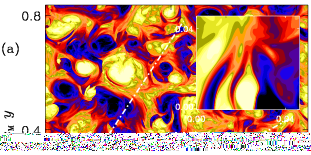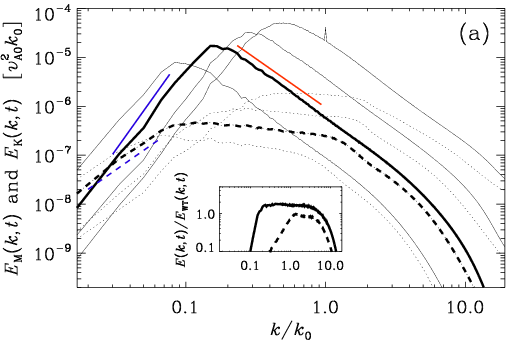Contours of (a) Bz(x,y) and (b) uz(x,y). The insets show a zoom into the small square in the lower left corner.

## Inverse transfer in nonhelial turbulence

In the presence of magnetic helicity, inverse transfer from small to large scales is well known in magnetohydrodynamic (MHD) turbulence and has applications in astrophysics, cosmology, and fusion plasmas. Using high resolution direct numerical simulations of magnetically dominated self-similarly decaying MHD turbulence, we report a similar inverse transfer even in the absence of magnetic helicity. We compute for the first time spectral energy transfer rates to show that this inverse transfer is about half as strong as with helicity, but in both cases the magnetic gain at large scales results from velocity at similar scales interacting with smaller-scale magnetic fields. This suggests that both inverse transfers are a consequence of a universal mechanisms for magnetically dominated turbulence. Possible explanations include inverse cascading of the mean squared vector potential associated with local near two-dimensionality and the shallower k^2 subinertial range spectrum of kinetic energy forcing the magnetic field with a k^4 subinertial range to attain larger-scale coherence. The inertial range shows a clear k^{-2} spectrum and is the first example of fully isotropic magnetically dominated MHD turbulence exhibiting weak turbulence scaling.(a) Magnetic (solid lines) and kinetic (dashed lines) energy spectra for Run~A at times t/τA=18, 130, 450, and 1800; the time t/τA=450 is shown as bold lines. The straight lines indicate the slopes k4 (solid, blue), k2 (dashed, blue), and k-2 (red, solid). (b) Same for Run~B, at t/τA==540, 1300, and 1800, with t/τA==1300 shown as bold lines. The insets show EM and EK compensated by EWT. References:
Brandenburg, A., Kahniashvili, T., & Tevzadze, A. G.: 2015, ``Nonhelical inverse transfer of a decaying turbulent magnetic field,'' Phys. Rev. Lett. 114, 075001